• 引导滤波/导向滤波原理与实现
2021-05-17 17:27:17

引导滤波/导向滤波是何凯明等人在2010年ECCV上提出来的，文章为《Guided Image Filtering》。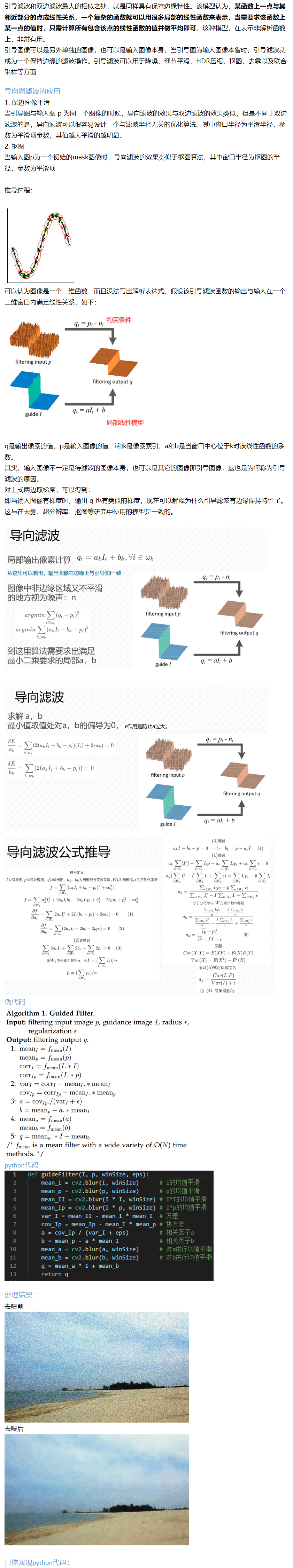import cv2
import numpy as np

input_fn=r"E:\study\python\python_code\code\CV\Guide_filter\pic\beach.jpg"

srcImg=srcImg/255.0
guidedImg=guidedImg/255.0
img_shape=np.shape(srcImg)

var_I=I_square_mean-np.multiply(I_mean,I_mean)
cov_I_P=I_mul_P_mean-np.multiply(I_mean,P_mean)

a=cov_I_P/(var_I+eps)
b=P_mean-np.multiply(a,I_mean)

dstImg=np.multiply(a_mean,guidedImg)+b_mean

return dstImg*255.0

img_shape=np.shape(srcImg)

dstImg=np.zeros(img_shape,dtype=float)

for ind in range(0,img_shape):
dstImg[:,:,ind]=my_guidedFilter_oneChannel(srcImg[:,:,ind],

dstImg=dstImg.astype(np.uint8)

return dstImg

def main():
print(np.shape(img))

dstimg=my_guidedFilter_threeChannel(img,img,9,0.01)
print(np.shape(dstimg))
cv2.imwrite('output.jpg',dstimg)
cv2.imshow('output',dstimg)
cv2.waitKey(0)

if __name__ == '__main__':
main()

更多相关内容
• 在图像处理上，导向滤波器（Guided Image Filter）是一种能使图像平滑化的非线性滤波器。与双边滤波器（Bilateral Filter）相同，这个滤波器同样能够在清楚保持图像边界的情况下，达到让图像平滑的效果。 但不同于...

# 前言

在图像处理上，导向滤波器（Guided Image Filter）是一种能使图像平滑化的非线性滤波器。与双边滤波器（Bilateral Filter）相同，这个滤波器同样能够在清楚保持图像边界的情况下，达到让图像平滑的效果。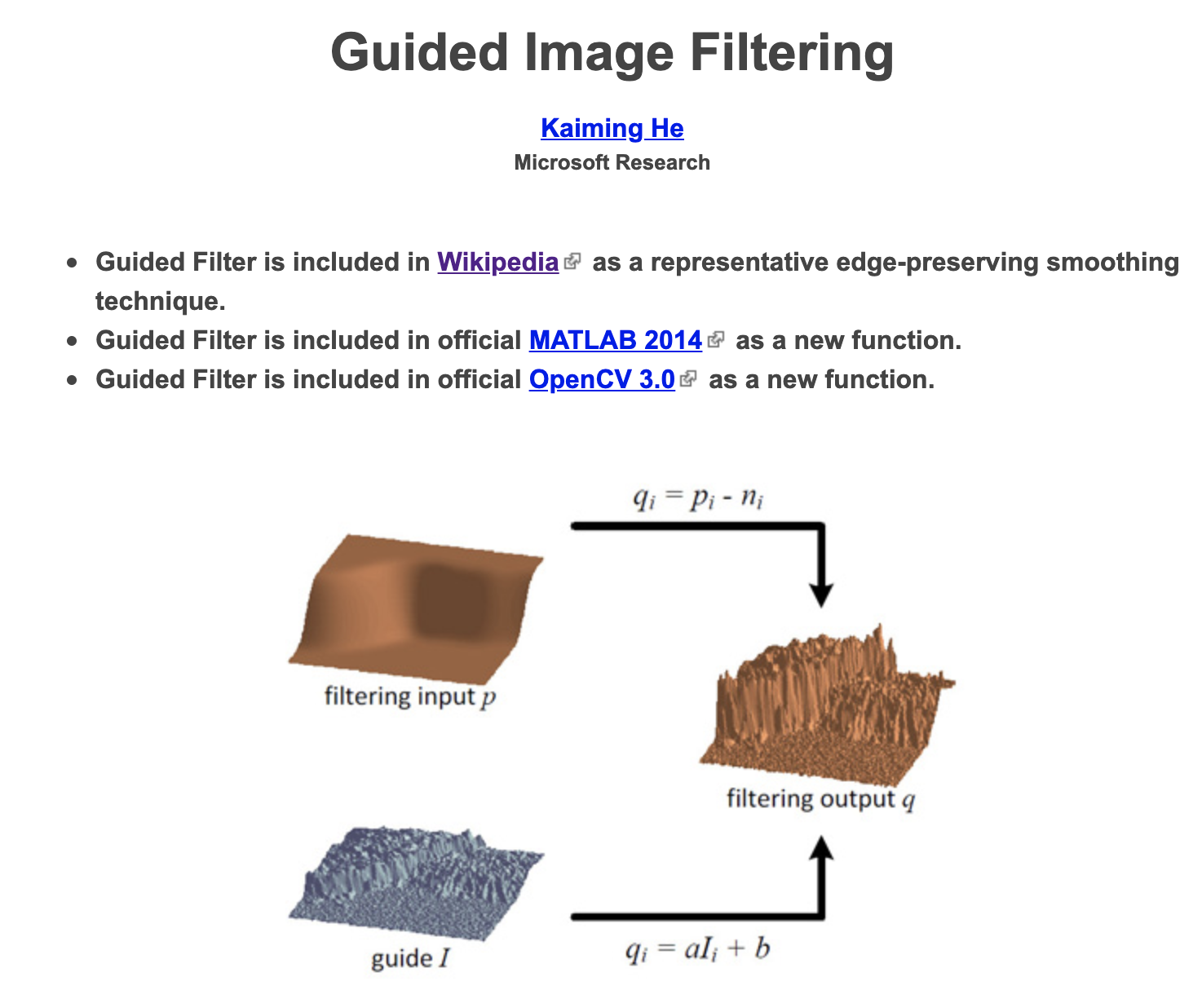但不同于双边滤波器，导向滤波器有两个优点：

1. 首先，双边滤波器有非常大的计算复杂度（O(N^2)），但导向滤波器因为并未用到过于复杂的数学计算，有线性的计算复杂度。

可以说，导向滤波相比双边滤波的两大优势就是速度快和不会有梯度反转。

实际的应用场景除了去噪平滑外，还可以用于细节加强（detail smoothing/enhancement，如“羽化”）、HDR compression、image matting/feathering、haze removal（去雾）、joint upsampling、深度图修整等功能。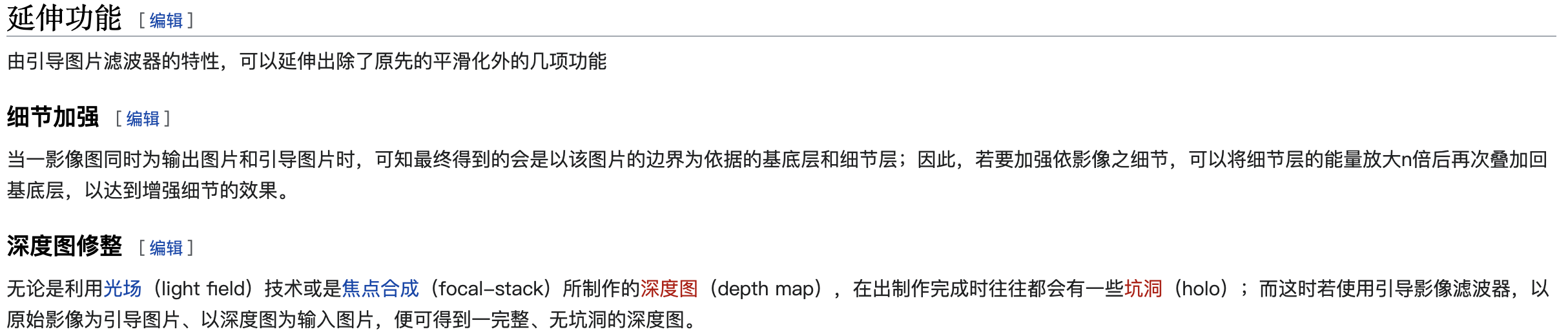# 原理

为了达到图像平滑去噪效果，首先定义输出的结果图是输入图减去噪声后的结果。同时，为了让输出图保持引导图的边界，将输出图定为引导图的线性组合。

可以说，导向滤波核心原理是假设导向图I与滤波结果输出图q符合局部（以像素 k k 为中心的 w k w_k 窗口内）线性模型：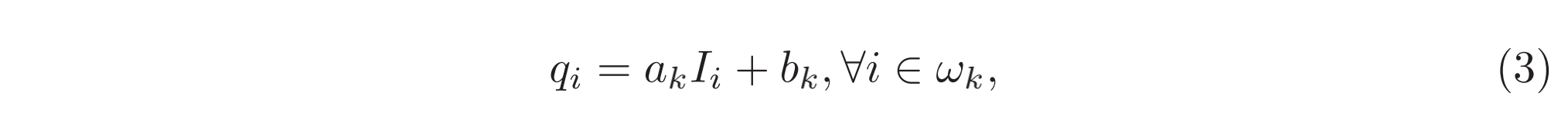局部线性模型（local linear model）保证了结果图与导向图的edge一致（ ∇ q = a ∇ I ∇q = a∇I ）。

为了得到线性系数，需要构建方程求解。论文采用的是最小化输出q与输入图p之间的差异，即最小化窗口内的代价函数：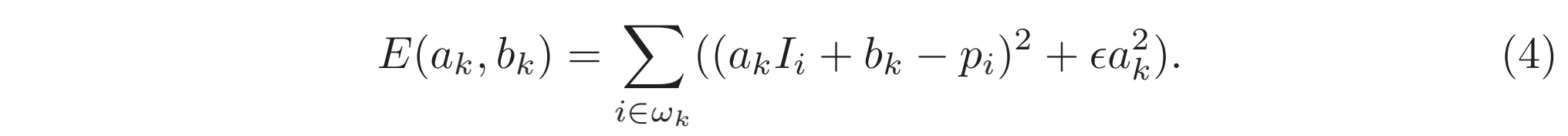其中 ϵ \epsilon 是防止 a k a_k 过大的正则化参数。

方程的解可以根据 linear regression 求得，细节见参考资料中推导：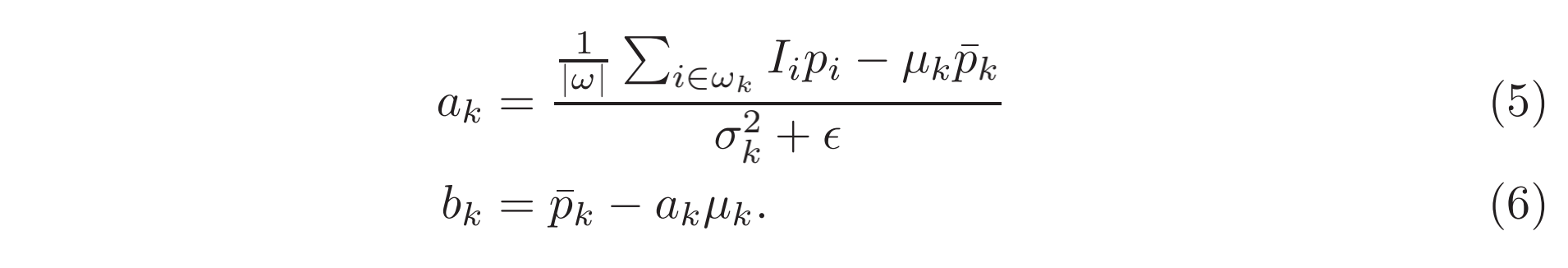其中， μ k \mu_k σ k 2 \sigma^2_k 是导向图I在窗口 w k w_k 内的均值和方差， ∣ w ∣ |w| 是窗口 w k w_k 内的像素数目， p ‾ k = 1 ∣ w ∣ ∑ i ∈ w k p i \overline{p}_k=\frac{1}{|w|}\sum_{i\in w_k}{p_i} 是窗口 w k w_k 内的均值。

基本上，根据得到的 a k a_k b k b_k 就可以计算得出窗口 w k w_k 内的每一个 q i q_i 。但是进一步考虑，由于每一个像素不一定只被一个窗口 w k w_k 所包含，例如九宫格情况下中心像素点就被9个3x3的 w k w_k 窗口包含。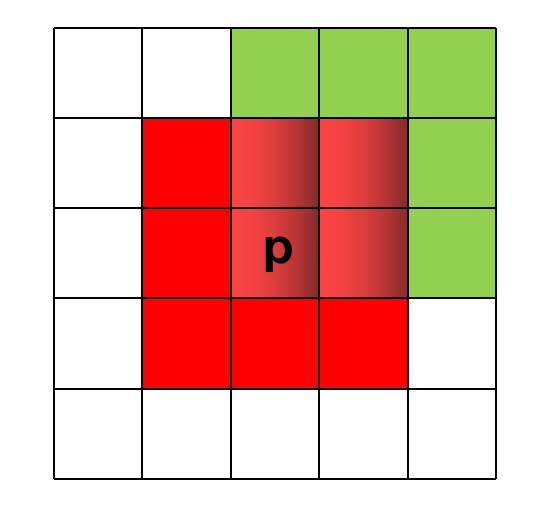所以最简单的方式则是对这9个 w k w_k 窗口得到的 q i q_i 做一个加权平均，得到的最终 q i q_i 才是真正的结果值。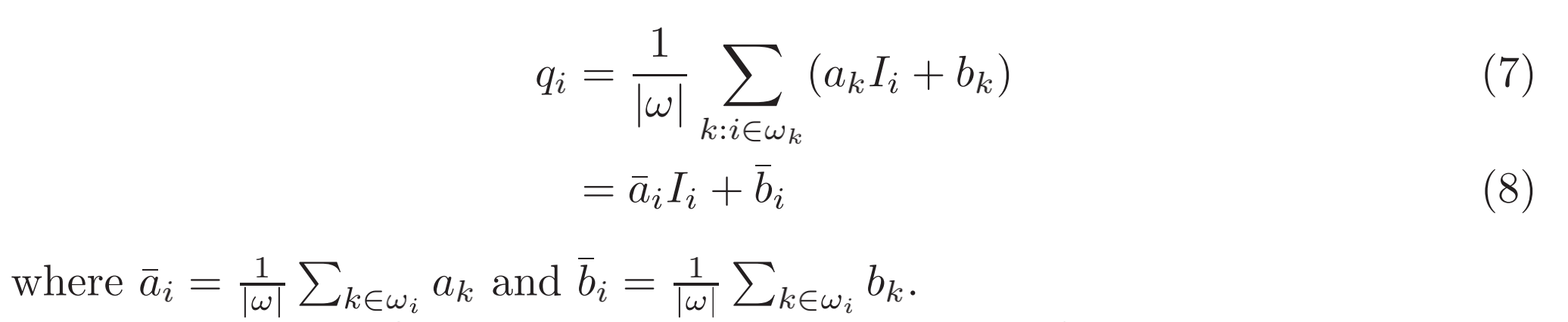经过对所有 q i q_i 的加权平均（实际上用的是均值滤波）， ∇ q ∇q 不再是 ∇ I ∇I 线性关系。但是由于 ( a ‾ i , b ‾ i ) (\overline a_i, \overline b_i) 是经过均值滤波得到，在导向图的强边界处，输出图的梯度会比导向图小。这种情况下可以认为 ∇ q ≈ a ‾ ∇ I ∇q \approx \overline a∇I ，表示导向图I边界的强变化还能被输出图q维持。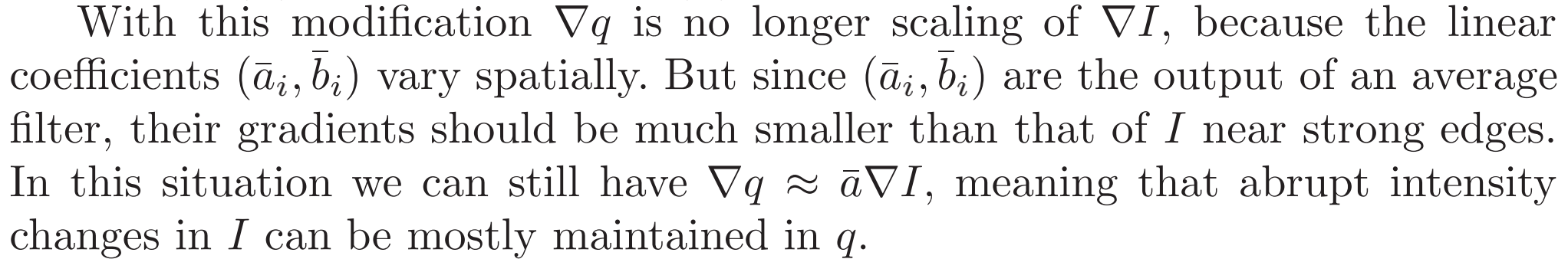算法伪码如下：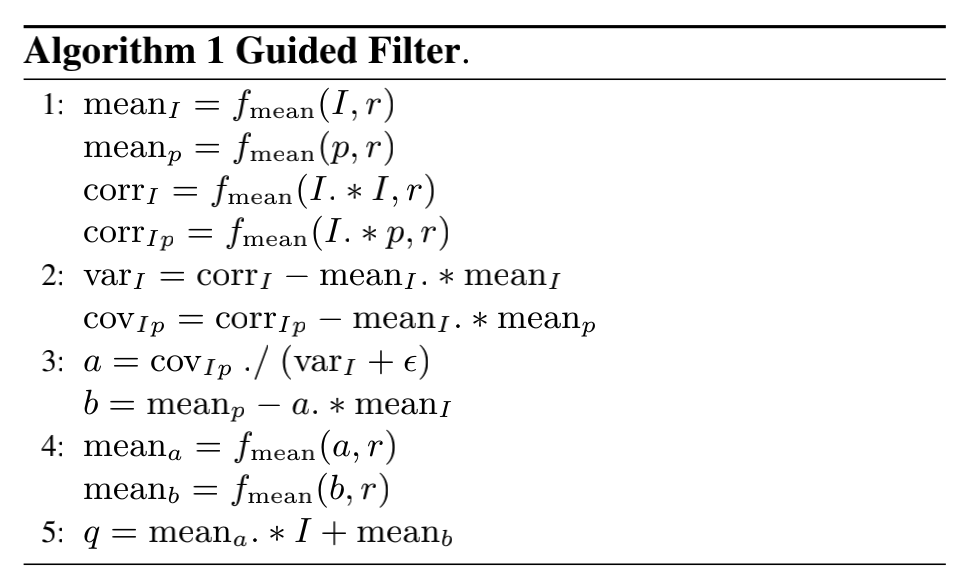其中， f m e a n ( ⋅ , r ) f_{mean}(·, r) 是半径为r的均值滤波器。

而方差和协方差定义如下：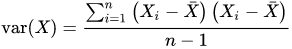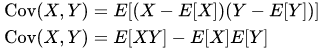对式子 (5) 进行变换，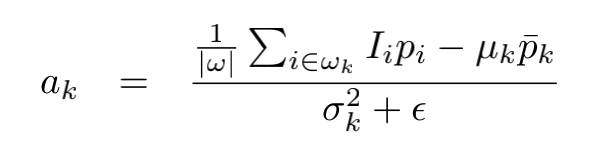则可以得到算法伪码中的：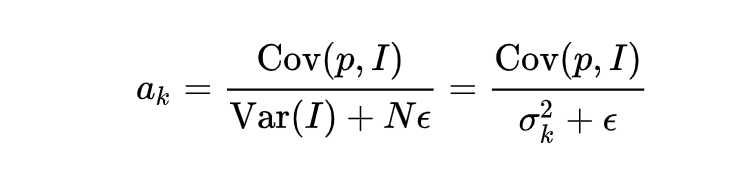特别说明：
通过参数 ϵ \epsilon 定义什么是“平坦区块(patch)”或“高变化区块”。若一个区块的方差远低于参数 ϵ \epsilon ，其通过滤波器后将被平滑；反之，方差远高于 ϵ \epsilon 的区块将被视为边界而被保留。

双边滤波中的范围方差(range variance)参数 σ r 2 \sigma _{r}^{2} 的功能和导向滤波的 ϵ \epsilon 相似。它们都定义了什么样的区块应该被平滑，而什么样的区块应该被保留。

# 实现

OpenCV中对导向滤波有CPU实现。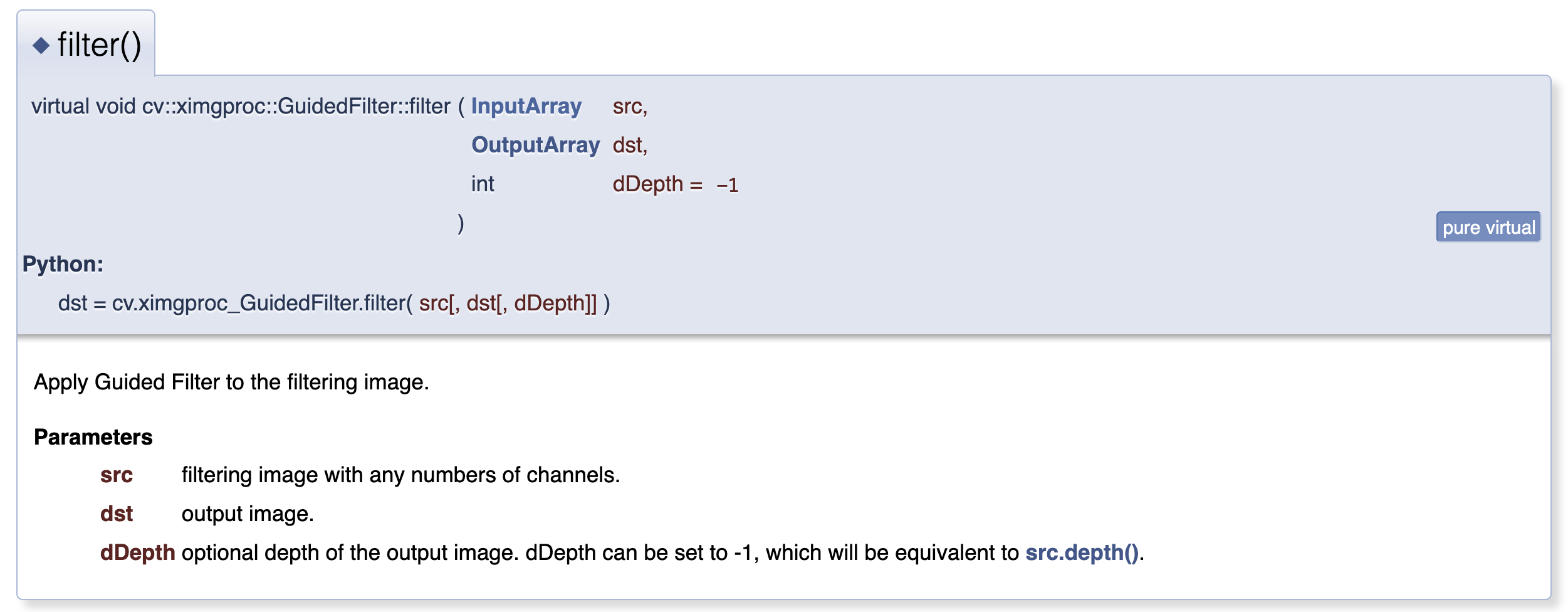核心代码如下：

void GuidedFilterImpl::filter(InputArray src, OutputArray dst, int dDepth /*= -1*/)
{
CV_Assert( !src.empty() && (src.depth() == CV_32F || src.depth() == CV_8U) );
if (src.rows() != h || src.cols() != w)
{
CV_Error(Error::StsBadSize, "Size of filtering image must be equal to size of guide image");
return;
}

if (dDepth == -1) dDepth = src.depth();
int srcCnNum = src.channels();

vector<Mat> srcCn(srcCnNum);
vector<Mat>& srcCnMean = srcCn;
split(src, srcCn);

if (src.depth() != CV_32F)
{
parConvertToWorkType(srcCn, srcCn);
}

vector<vector<Mat> > covSrcGuide(srcCnNum);
computeCovGuideAndSrc(srcCn, srcCnMean, covSrcGuide);

vector<vector<Mat> > alpha(srcCnNum);
for (int si = 0; si < srcCnNum; si++)
{
alpha[si].resize(gCnNum);
for (int gi = 0; gi < gCnNum; gi++)
alpha[si][gi].create(h, w, CV_32FC1);
}
runParBody(ComputeAlpha_ParBody(*this, alpha, covSrcGuide));
covSrcGuide.clear();

vector<Mat>& beta = srcCnMean;
runParBody(ComputeBeta_ParBody(*this, alpha, srcCnMean, beta));

parMeanFilter(beta, beta);
parMeanFilter(alpha, alpha);

runParBody(ApplyTransform_ParBody(*this, alpha, beta));
if (dDepth != CV_32F)
{
for (int i = 0; i < srcCnNum; i++)
beta[i].convertTo(beta[i], dDepth);
}
merge(beta, dst);
}



具体文件参考GitHub的OpenCV Contrib包实现

GPU版导向滤波实现参考GitHub - TracelessLe/pybind11_guidedfilter_cuda

cv::cuda::GpuMat GuidedFilterMono::filterSingleChannel(const cv::cuda::GpuMat &p, cv::cuda::Stream &stream) const {
cv::cuda::GpuMat mean_p, mean_Ip, cov_Ip;
box_filter->apply(p, mean_p, stream);
cv::cuda::multiply(I, p, mean_Ip, 1, -1, stream);
box_filter->apply(mean_Ip, mean_Ip, stream);
cv::cuda::multiply(mean_I, mean_p, cov_Ip, 1, -1, stream);
cv::cuda::subtract(mean_Ip,
cov_Ip,
cov_Ip,
cv::noArray(),
-1,
stream); // this is the covariance of (I, p) in each local patch.

cv::cuda::GpuMat a, b;
cv::cuda::add(var_I, cv::Scalar(eps), a, cv::noArray(), -1, stream);
cv::cuda::divide(cov_Ip, a, a, 1, -1, stream); // Eqn. (5) in the paper;

cv::cuda::multiply(a, mean_I, b, 1, -1, stream);
cv::cuda::subtract(mean_p, b, b, cv::noArray(), -1, stream); // Eqn. (6) in the paper;

box_filter->apply(a, a, stream);
box_filter->apply(b, b, stream);

cv::cuda::multiply(a, I, a, 1, -1, stream);
cv::cuda::add(a, b, a, cv::noArray(), -1, stream);

return a;
}


# 扩展讨论

（1）相比双边滤波，导向滤波有速度快和避免梯度反转等优势。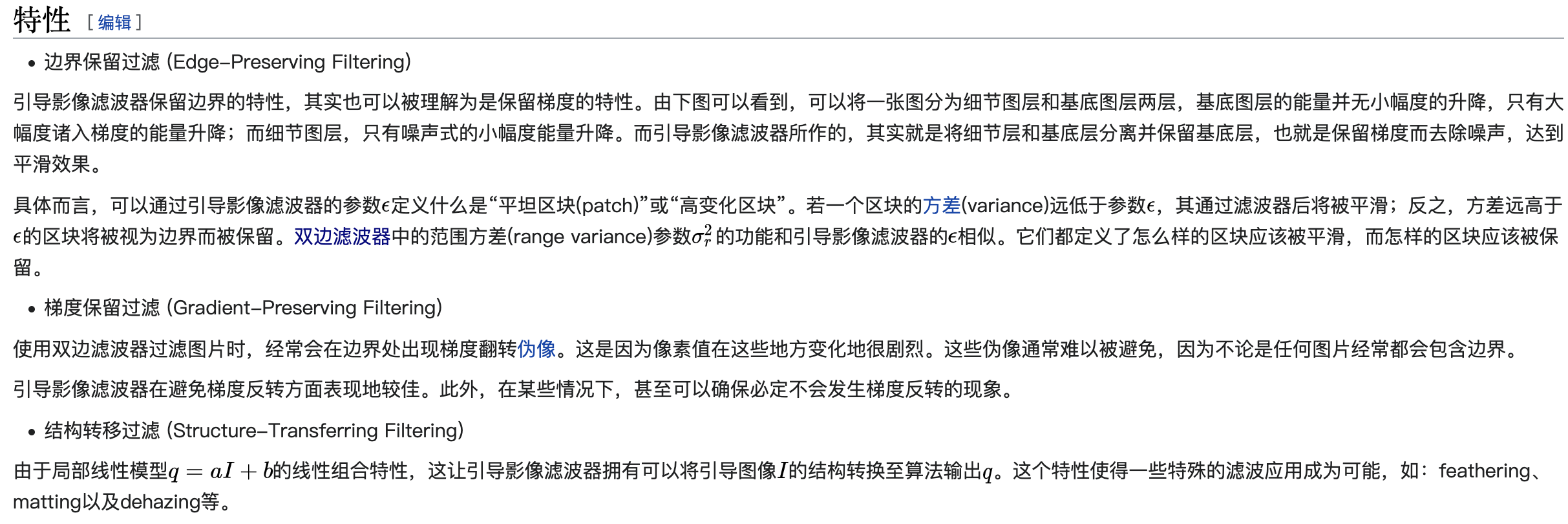（2）基于原始的导向滤波算法引入resize得到的Fast导向滤波能够将时间复杂度从O(N)降到O(N / r^2)，同时保证滤波结果图像质量损失不大。其中r是resize（或称之为scale）的倍数。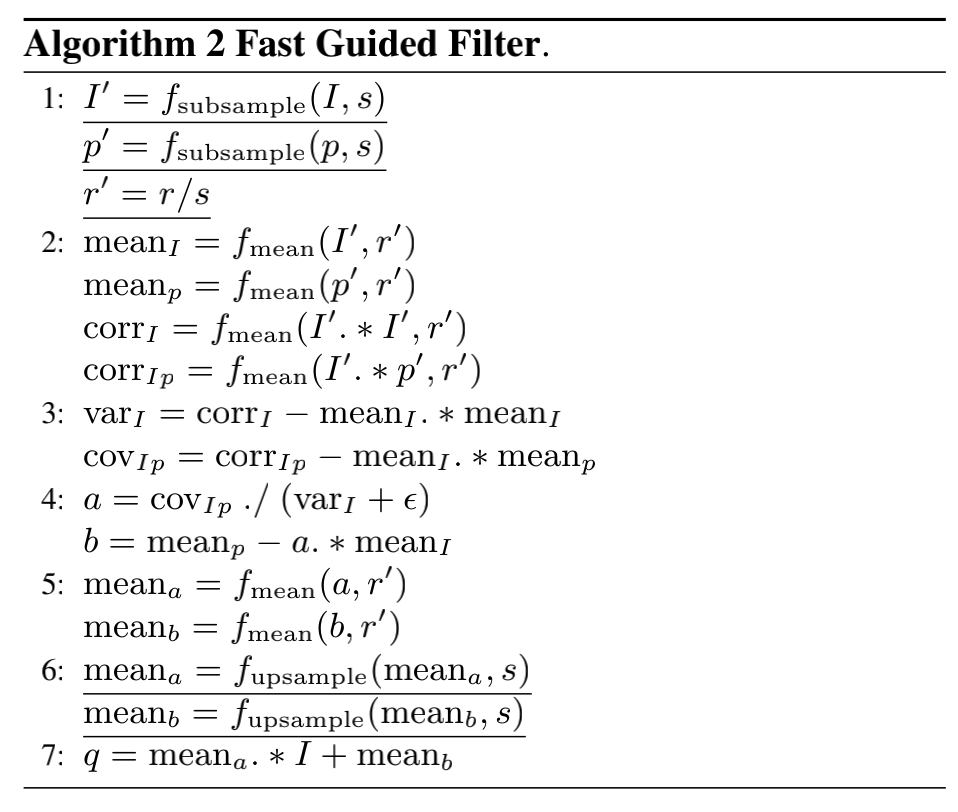# 参考资料

展开全文计算机视觉 opencv
• 基于导向滤波的work论文，写的非常详细，希望能够帮助到广大科研人员
• 二、原理 引导滤波的思想是： 认为输出图像与引导图像在 小范围 内是 线性关系 ，将起伏不定的连续像素看成一段段的小线性函数，每一小段的公式如下： qi=akIi+bkq_{i}=a_{k}I_{i}+b_{k}qi​=ak​Ii​+bk​ 其中i是...

## 一、性质

引导滤波的特性是：

当引导图比较陡峭时，输出图像应随着引导图变化而变化，当引导图较为缓和时，输出图像应接近输入图像。

## 二、原理

引导滤波的思想是：

认为输出图像与引导图像在 小范围 内是 线性关系 ，将起伏不定的连续像素看成一段段的小线性函数，每一小段的公式如下：

q i = a k I i + b k q_{i}=a_{k}I_{i}+b_{k}

其中i是像素坐标，I为引导图像素值，q为线性函数拟合的输出图像，ak、bk是线性函数的两个参数，我们将这个线性函数的适用范围记为k。

也就是说，输出图像要跟着导向图的趋势走，在一个小范围内，如果导向图是平坦的，那么a趋近于0，输出图像q应等于b，如果导向图较为陡峭，a很大，则输出图像q应接近a*I

（当导向图为原图时，a*I相当于原图乘以一个系数，当a=1、b=0，那直接就是原图）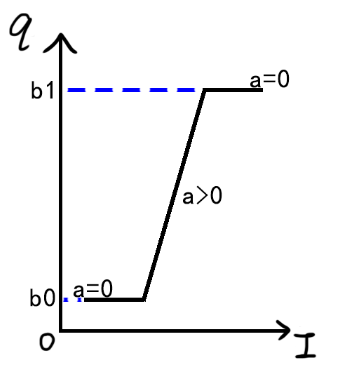## 三、损失函数

由上一节的线性公式得知，如果变化是陡峭的，斜率是大的，那系数a可以很容易通过初一的知识得到：

a = Δ q Δ I a=\frac{\Delta q }{\Delta I }

但如果变化是平坦的，b又该如何得到呢？这时候就该原图登场了，由第一节的概念可知，平坦时输出图像应接近原图，因此可写作下面的式子：

q i − p i = n i q_{i}-p_{i}=n_{i}

这里n代表原图与输出的误差。

为了成功还原原图，我们需要最小化误差n，因此常用的损失函数如下（令E最小）：

E ( a k , b k ) = ∑ i ∈ w k ( q i − p i ) 2 E(a_{k},b_{k})=\sum_{i\in w_{k}}^{}(q_{i}-p_{i})^{2}

这里的wk代表滤波窗口的范围，将q展开：

E ( a k , b k ) = ∑ i ∈ w k ( a k I i + b k − p i ) 2 E(a_{k},b_{k})=\sum_{i\in w_{k}}^{}(a_{k}I_{i}+b_{k}-p_{i})^{2}

分别令E对a和b求导，得到a与b的解之后，我们的线性函数能够较为完美的拟合原图，以至于当引导图为原图时，输出完全就是原图。如果最终的结果是这样，那就很难做到保边缘滤波了。因为已经完全没有了滤波。

通常，我们还是希望能够控制这种拟合的程度，在机器学习中，为了防止过拟合，需要在损失函数中引入正则化，在这里也可以同样操作：

E ( a k , b k ) = ∑ i ∈ w k ( ( a k I i + b k − p i ) + ϵ a k 2 ) 2 E(a_{k},b_{k})=\sum_{i\in w_{k}}^{}((a_{k}I_{i}+b_{k}-p_{i})+\epsilon a_{k}^{2})^{2}

其中 ϵ \epsilon 叫做正则化系数，可以调节拟合程度，当取值为0时，平坦区域就更接近原图。
用自变量前面的参数来防止过拟合的原因，大体可以解释如下：

拟合过程中通常都倾向于让权值尽可能小，最后构造一个所有参数都比较小的模型。因为一般认为参数值小的模型比较简单，能适应不同的数据集，也在一定程度上避免了过拟合现象。可以设想一下对于一个线性回归方程，若参数很大，那么只要数据偏移一点点，就会对结果造成很大的影响；但如果参数足够小，数据偏移得多一点也不会对结果造成什么影响，专业一点的说法是『抗扰动能力强』。
https://blog.csdn.net/jinping_shi/article/details/52433975
不懂就记住好了。。

对加入了正则化项的公式求ak、bk的偏导，令导数为0，可求得：

b k = p k ˉ − a k I k ˉ b_{k}=\bar{p_{k}}-a_{k}\bar{I_{k}}

a k = p k I k ‾ − p k ‾ I k ‾ σ 2 + ϵ a_{k}=\frac{\overline{p_{k}I_{k}}-\overline{p_{k}}\overline{I_{k}}}{\sigma ^{2}+\epsilon }

公式中出现了均值和方差，这都是在求导过程中凑出来的。

至此，输出图像可直接由 q i = a k I i + b k q_{i}=a_{k}I_{i}+b_{k} 算出。

## 四、保边缘滤波

当引导图为原图时，导向滤波是一个很好的保边缘滤波器，怎么理解呢？要从ak和bk的公式说起。

根据第三节最后的公式，我们令Ik等于pk，可得：

a k = p k 2 ‾ − p k ‾ 2 σ 2 + ϵ = σ 2 σ 2 + ϵ a_{k}=\frac{\overline{p_{k}^{2}} - \overline{p_{k}}^{2}}{\sigma ^{2}+\epsilon } =\frac{\sigma ^{2}}{\sigma ^{2}+\epsilon }

b k = ( 1 − a k ) p k ‾ b_{k}=(1-a_{k})\overline{p_{k}}

请出关键公式(I已经替换为p)：

q i = a k p i + b k q_{i}=a_{k}p_{i}+b_{k}

在图像的边缘处，方差较大，ak趋于1，bk趋于0，输出图像等于输入图像，实现保边缘

在图像的平坦/纹理处，方差较小，ak较小，输出更接近bk，而bk趋于图像均值 p k ‾ \overline{p_{k}} ，实现均值滤波

滤波的程度则由 ϵ \epsilon 控制，其为0时，输出永远等于输入图像，与第三节的结论相同。

展开全文图像处理
• 导向滤波原理（Guided Filter）

# 导向滤波原理（Guided Filter）

## 简介

导向滤波（Guided Fliter）显式地利用 guidance image 计算输出图像，其中 guidance image 可以是输入图像本身或者其他图像。导向滤波比起双边滤波来说在边界附近效果较好；另外，它还具有 O(N) 的线性时间的速度优势。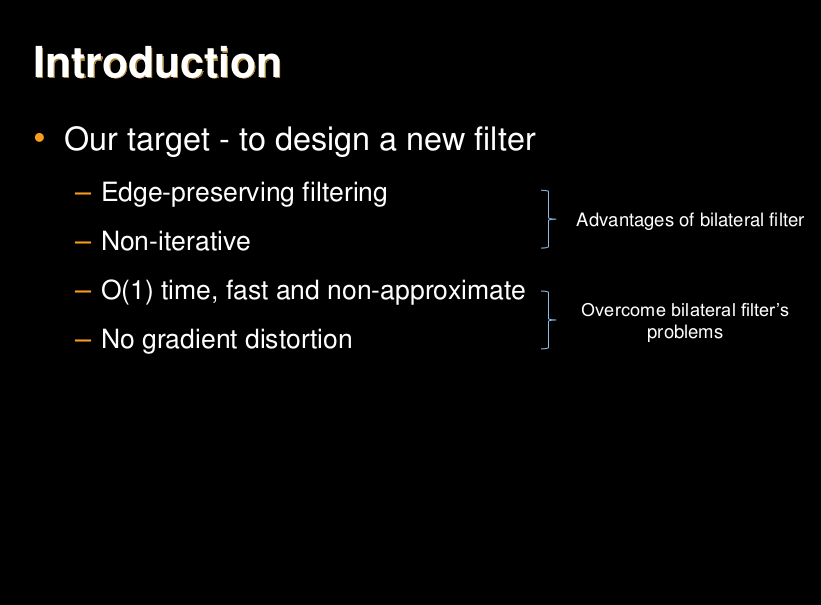## 相关工作

1. Explicit Weighted-Average Filters（显式加权平均滤波器）

双边滤波可以在平滑的过程中保持边缘，但是会出现不希望的 gradient reversal 的 artifact。原因在于如果一个 pixel 周围有较少的相似的 pixel，那么Gaussian weighed average 就会不稳定。 另外就是双边滤波的效率问题，brute-force 实现双边滤波需要 O(Nr^2) 的时间，后来又有文章提出了O(Nlogr) 和 O(N) 的实现方法。但是这些加速方法需要coarse sampling，从而在Nyquist condition被严重破坏的情况下，牺牲了质量。其他此类滤波器还有 Edge-Avoiding Wavelets，Domain Transform Filter。

1. Implicit Weighted-Average Filters （隐式加权平均滤波器）

优化损失函数，求解线性系统的方法等价与隐式图像滤波。尽管 optimization-based 方法一般可以得到高质量的结果，但是求解线性系统较为费时间。已经有人证明这些隐式的滤波器和显式的是相关联的，显式的滤波器也可以写成求解矩阵问题的形式。

1. Nonaverage Filters （非均值滤波器）

保边滤波可以不用平均的方法，如中值滤波，可以看成是一个局部的直方图滤波器。 其余还有 Total-Variation filters。非均值滤波器通常都是计算量较大的。

## 导向滤波（Guided Filter）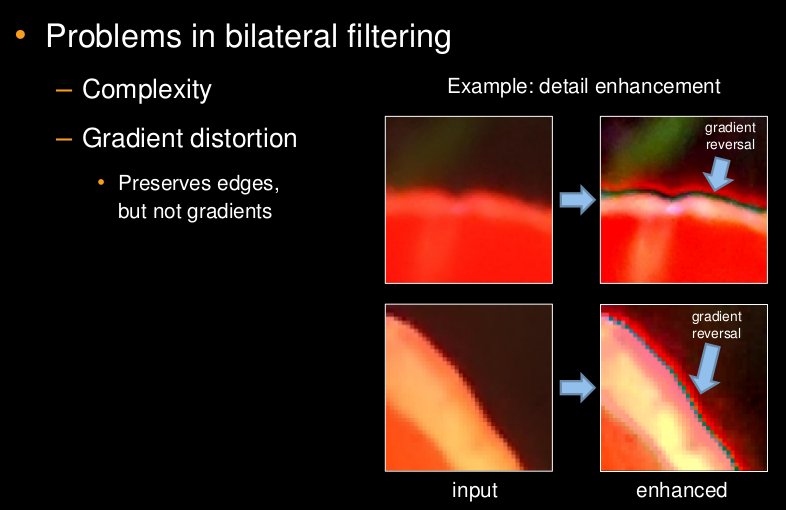而导向滤波可以避免这一缺点。基本原理如下：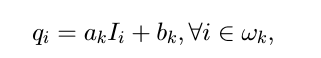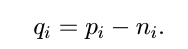其中，p为输入图像，I 为导向图，q 为输出图像。在这里我们认为输出图像可以看成导向图I 的一个局部线性变换，其中k是局部化的窗口的中点，因此属于窗口 ωk $\omega_k$ 的pixel，都可以用导向图对应的pixel通过（ak,bk）的系数进行变换计算出来。同时，我们认为输入图像 p 是由 q 加上我们不希望的噪声或纹理得到的，因此有p = q + n 。

接下来就是解出这样的系数，使得p和q的差别尽量小，而且还可以保持局部线性模型。这里利用了带有正则项的 linear ridge regression（岭回归）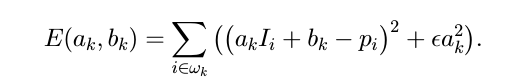求解以上方程得到a和b在局部的值，对于一个要求的pixel可能含在多个window中，因此平均后得到：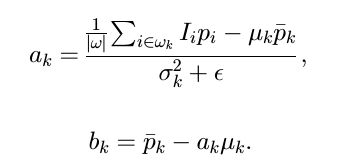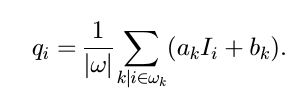最后得到的算法为：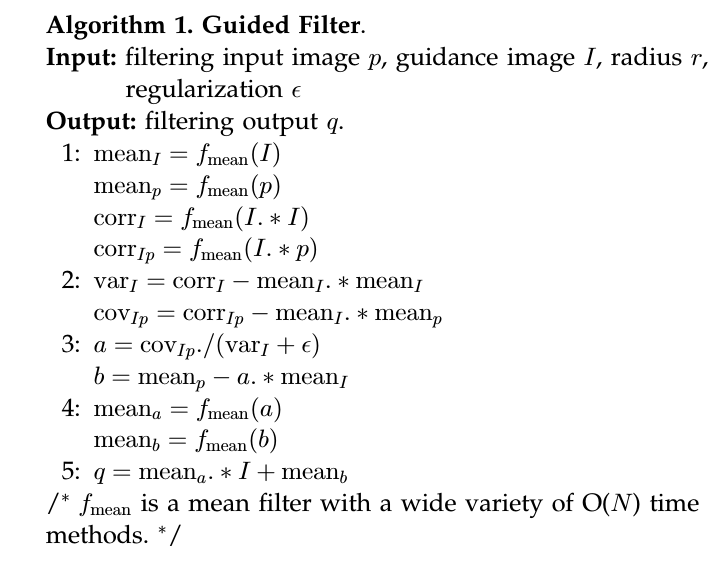其中的fmean是指具有窗口半径r的均值滤波。下面说明为何其可以实现平滑保边的效果。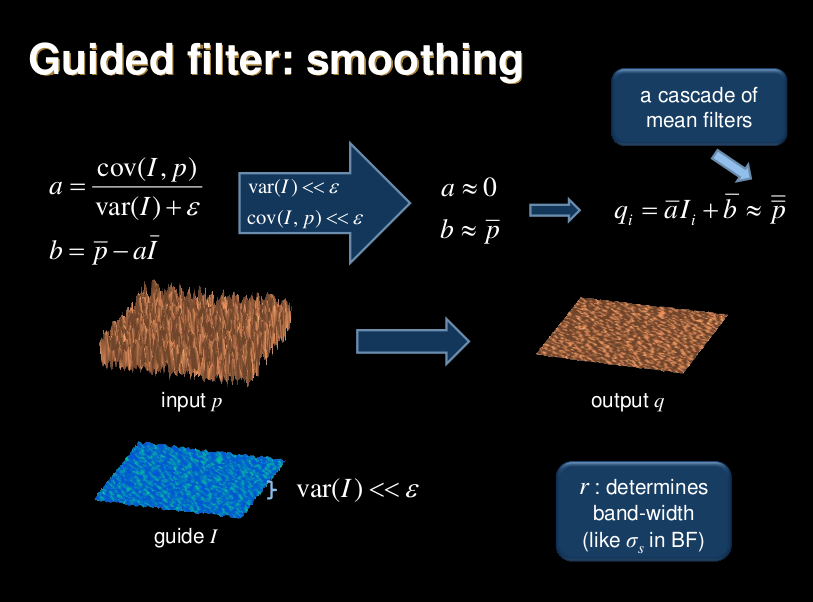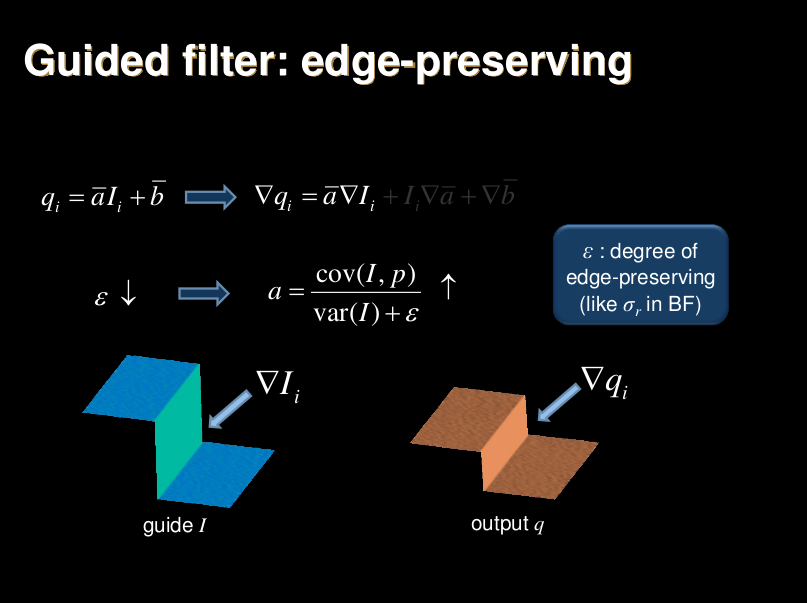可以看出，如果 I 和 p 相同，那么每个窗口内的 ak 就是输入图像的方差比上方差加 ϵ $\epsilon$ ，b 等于(1 - ak)乘以该窗口内的原图像的平均值。

• 如果方差较小，即是一个平滑区域的话，那么方差较小约等于0，则ak约等于方差除以epsilon，因此 ak 约等于 0 。那么bk约等于1, 那么qi = mean(p)，这样相当于说这个pixel在该窗口内的输出值相当于在这个窗口进行了均值平滑，而考虑到pixel属于多个窗口，如果这是一个平滑区域，那么就相当与多个均值平滑滤波器的级联。
• 如果方差大，即是边缘的话，ak 就约等于 1 （epsilon很小），因此，b 就约等于0。相当于输出等于输入只是乘以一个约等于1的系数，这样可以保证梯度的比例关系不变。

为了展示GF和BF的效果的相似性，将同一图像经过GF和BF滤波后，计算两个输出的“PSNR”，（It is often considered as visually insensitive when the PSNR >= 40 dB）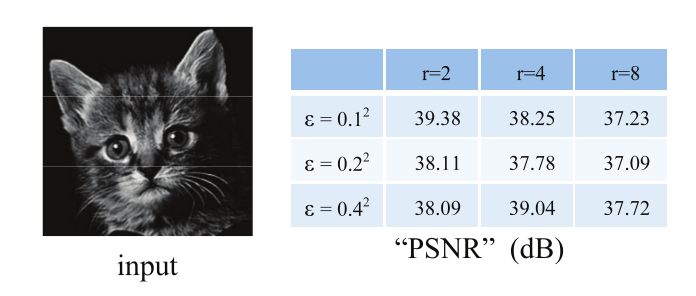下面展示的是可以改善BF梯度翻转的缺陷。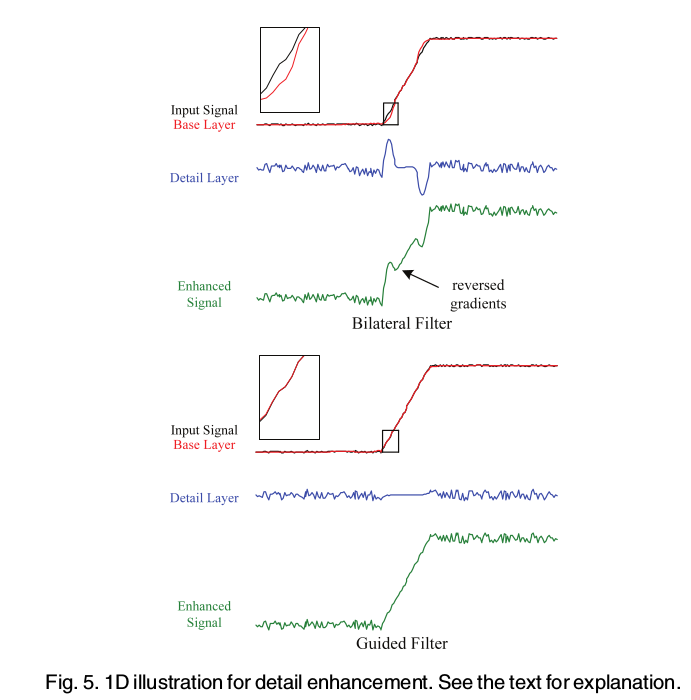除了平滑保边滤波以外，GF还可以作为结构转移滤波。（Interestingly, the guided filter is not simply a smoothing filter. Due to the local linear model of q = aI+b, the output q is locally a scaling (plus an offset) of the guidance I. This makes it possible to transfer structure from the guidance I to the output q, even if the filtering input p is smooth），因此可以用于feathering/matting 以及 dehazing 等一系列的应用。

文中还提到一个计算fmean时候的加速算法，由于计算每个像素点都要对周围2r+1个点进行求和（1D情况），但是相邻内两个需要计算的像素点实际上只有一个求和的加数有差别，即右边的pixel只是将左边的pixel已经求好的和减去求和时候的最左边一个数，再加上最右边的右边的数值。例如，如果 r=2 求 fmean(5) = f(3) + f(4) + f(5) + f(6) + f(7) ，而fmean(6) = f(4) + f(5) + f(6) + f(7) + f(8) ，因此 fmean(6) = fmean(5) - f(3) + f(8) 。这样计算当r比较大的时候可以显著减少运算量，避免了重复求和。算法如下：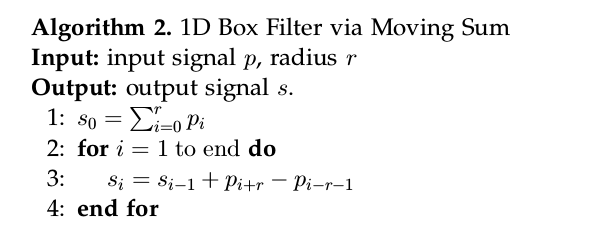ps : GF 还有更高的提速的算法，通过降采样求出a和b，在升采样回原尺寸，并用来对 I 线性变换。这样时间可以由 O(N) 降低到 O(N/s^2) ，其中s是采样率。

算法如下：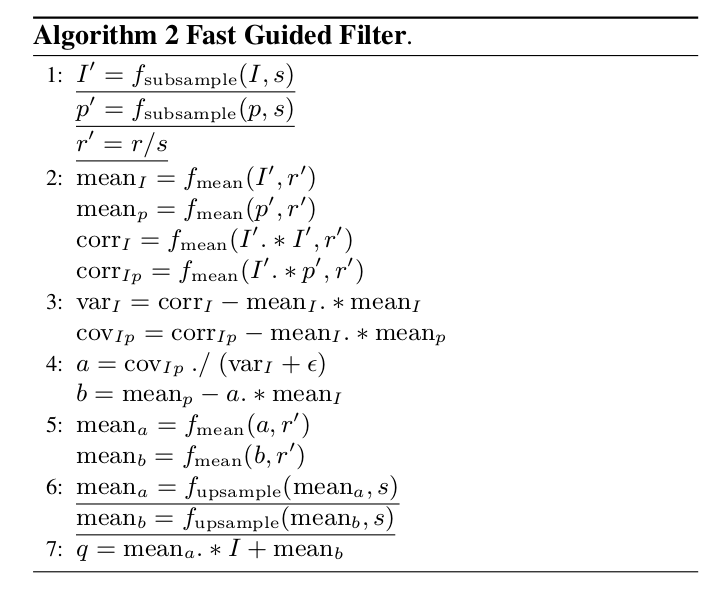THE END

reference :

 K. He, J. Sun, and X. Tang. Guided image filtering. In ECCV, pages 1–14. 2010.

 K. He, J. Sun, and X. Tang. Guided image filtering. TPAMI, 35(6):1397–1409, 2013

 He K, Sun J. Fast Guided Filter[J]. Computer Science, 2015.

ECCV 2010 presentation slides

2017/12/22 Fri 14:34

展开全文filter
• 参考： 知乎：导向滤波原理（Guided Filter） CSDN博客：双边滤波与引导滤波
• 一、双边滤波双边滤波很有名，使用广泛，简单的说就是一种同时考虑了像素空间差异与强度差异的滤波器，因此具有保持图像边缘的特性。先看看我们熟悉的高斯滤波器：其中W是权重，i和j是像素索引，K是归一化常量。公式...
• 简介导向滤波（Guided Fliter）显式地利用 guidance image 计算输出图像，其中 guidance image 可以是输入图像本身或者其他图像。导向滤波比起双边滤波来说在边界附近效果较好；另外，它还具有 O(N) 的线性时间的...
• 在图像滤波算法中，导向滤波、双边滤波、最小二乘滤波并称三大保边滤波器，他们是各向异性滤波器。相对于常见的均值滤波、高斯滤波等各向同性滤波器，他们最大的特点是在去除噪声的同时，能最大限度保持边缘不被平滑...
• 本文为转载，原博客地址：https://blog.csdn.net/fu_shuwu/article/details/785085761. 导向滤波简介导向滤波是何凯明在学生时代提出的一个保边滤波（edge-preserving smoothing）算法。何凯明在cv圈应该算是名人了...
• Kaiming He的导向滤波的matlab代码。
• ## 导向滤波(Guided Filter)公式详解

万次阅读 多人点赞 2019-05-05 14:13:28
Guided Filter 导向滤波 Guided Image Filtering - 何恺明 2009   导向滤波（Guided Filtering）和双边滤波（BF）、最小二乘滤波（WLS）是三大边缘保持（Edge-perserving）滤波器。当然，引导滤波的功能不仅仅是...保边滤波
• 导向滤波的一般表达方式 qi=∑jWij(I)pj q_{i}=\sum_{j} W_{i j}(I) p_{j} qi​=j∑​Wij​(I)pj​ 其中qqq表示输出，ppp表示输入，III表示导向图。 先验假设 假设在局部范围内，输出图与导向图的关系可以用一个线性...计算机视觉 算法
• 1. 导向滤波算法 导向图像(Guidance Image) I，滤波输出图像(Filtering Input Image) p，均值平滑窗口半径 r，正则化参数 e。 2. 快速导向滤波算法 通过下采样减少像素点，计算mean_a & mean_b后进行上采样恢复...
• ## OpenCV—Python 导向滤波

千次阅读 多人点赞 2018-11-19 15:23:13
一、导向滤波原理 导向滤波是使用导向图像作为滤波内容图像，在导向图像上实现局部线性函数表达，实现各种不同的线性变换，输出变形之后的导向滤波图像。根据需要，导向图像可以跟输入图像不同或者一致。 公式及...
• 我主要说的导向滤波其中的引导图选择问题. 百度百科的定义 : 导向图滤波是一种图像滤波技术 ，通过一张引导图G（导向图），对目标图像P（输入图像）进行滤波处理，使得最后的输出图像大体上与目标图像P相似，但是...
• 导向滤波磨皮的对应文献为：Guided Image Filtering，这个算法速度极其之快，比其他的保边缘磨皮算法都快，甚至快上好几倍。这个算法最初来自于何明凯的图像去雾算法中，现在已然被应用封装与matlab图像处理函数库中...图像处理
• 导向滤波算法原理 原理可以看博主：白马负金羁 的文章导向滤波(Guided Filter)的解析与实现，对原理解释十分通俗易懂。 导向滤波： 1.实现的伪代码： 导向图像(Guidance Image) I，滤波输出图像(Filtering ...opencv python
• 导向滤波（Guided Fliter）显式地利用 guidance image 计算输出图像，其中 guidance image 可以是输入图像本身或者其他图像。导向滤波比起双边滤波来说在边界附近效果较好；另外，它还具有 O(N) 的线性时间的速度...
• 何恺明的暗原色先验图像去雾代码 用导向滤波代替软图像抠图来计算透射率分布
• 1.介绍提到导向滤波，首先想到的是“何恺明”，他的确是真大神，在图像领域，是中国人的骄傲，深度学习流行的时候，也是提出各种新算法，比如ResNets...2.导向滤波至于导向滤波原理推导，我就不介绍了，直接把算法...
• 导向滤波(Guided Filter) 导向滤波(Guided Filter) 1. 前言 2. 导向滤波(Guided Filter)描述 3. 代码实现 4. 参考 1. 前言 导向滤波(Guided Filter)是何恺明2010年发表在ECCV的文章《Guided Image Filtering...图像处理 图像增强 计算机视觉 人工智能
• ## 导向滤波详解

万次阅读 2018-05-23 16:34:47
现在从一个最简单的情形来开始...滤波之后的图像为qq，如下图所示，图像qq中第ii个像素是由图像pp中以第ii个像素为中心的一个窗口ww中的像素确定的。具体而言，在简单平滑中，图像qq中第ii个像素是由图像pp中以第...
• 简介：导向滤波（Guided Fliter）显式地利用 guidance image 计算输出图像，其中 guidance image 可以是输入图像本身或者其他图像。导向滤波比起双边滤波来说在边界附近效果较好；另外，它还具有 O(N) 的线性时间的...图像处理
• 1、原理 高斯滤波是以距离为权重，设计滤波模板作为滤波系数，只考虑了像素间的空间位置上的关系，因此滤波的结果会丢失边缘的信息。 高斯滤波的缺陷如下图所示：平坦区域正常滤波，图像细节没有变化，而在突变的......